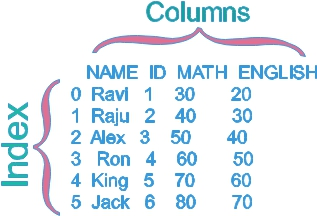Pandas DataFrame Attributes

 T Transpose , Changing Columns and indexes at value at input row , column columns Name of the Columns as List dtypes Data types of columns empty Checking if DataFrame is empty iat Data at position ( integer based )given as row and column iloc Data at (label based ) index Details on row based is_copy Return the copy ( deprecated) ix Data based on row and column ( deprecated) loc Data based on (label based ) Position ndim Dimension ( axis ) of the DataFrame shape Number of rows and column as tuple size Number of elements in DataFrame style Associated HTML style values Numpy representation of the DataFrame
List all attributes and methods of Pandas module by using dir()
import pandas as pd
print(dir(pd))
Use this code for examples of all sample attributes shown below.
import pandas as pd
my_dict={'NAME':['Ravi','Raju','Alex','Ron','King','Jack'],
'ID':[1,2,3,4,5,6],'MATH':[30,40,50,60,70,80],'ENGLISH':[20,30,40,50,60,70]}
my_data = pd.DataFrame(data=my_dict)
print(my_data)We can print the output here
NAME  ID  MATH  ENGLISH
0  Ravi   1    30       20
1  Raju   2    40       30
2  Alex   3    50       40
3   Ron   4    60       50
4  King   5    70       60
5  Jack   6    80       70
Above code will be used to check different attributes

TT :Transpose , Changing Columns and indexes
print(my_data.T)
0     1     2    3     4     5
NAME     Ravi  Raju  Alex  Ron  King  Jack
ID          1     2     3    4     5     6
MATH       30    40    50   60    70    80
ENGLISH    20    30    40   50    60    70

atat : value at by row, column pair
print(my_data.at[3,'ENGLISH']) # 50

columnscolumns: Name of the Columns
print(my_data.columns)
Output
Index(['NAME', 'ID', 'MATH', 'ENGLISH'], dtype='object')

dtypesdtypes : dtypes of used DataFrame
print(my_data.dtypes)
Output
NAME       object
ID          int64
MATH        int64
ENGLISH     int64
dtype: object

emptyempty : The DataFrame empty or not ( True or False )
print(my_data.empty) # False

iatiat : Value at position at rows and columns as integers ( inputs ).
print(my_data.iat[2,3]) # 40

ilociloc Values at different Positions , More on iloc

indexindex : Details on row labels
print(my_data.index) # RangeIndex(start=0, stop=6, step=1)

is_copyis_copy : deprecated

ixix : deprecated , position based on row and column
print(my_data.ix[2,'MATH']) # 50

locloc : Values , More on loc

ndimndim : array dimensions or axes
print(my_data.ndim) #2

shapeshape : Tuple giving dimension of DataFrame as ( rows, columns )
print(my_data.shape) # (6,4)

sizesize : Number of elements in the DataFrame
print(my_data.size) #24

stylestyle : Associated html style
print(my_data.style)

valuesvalues : All values of the DataFrame without axes labels. Numpy representation of the DataFrame.
print(my_data.values)
Output is here
[['Ravi' 1 30 20]
['Raju' 2 40 30]
['Alex' 3 50 40]
['Ron' 4 60 50]
['King' 5 70 60]
['Jack' 6 80 70]]

Subscribe

* indicates required
Subscribe to plus2netplus2net.com